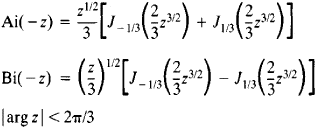# Airy Function

(redirected from Airy equation)

## Airy function

[¦er·ē ¦fəŋk·shən]
(mathematics)
Either of the solutions of the Airy differential equation.

## Airy Function

either of the functions Ai(z) and Bi(z), which are solutions of the second-order differential equation

W″zW = 0

where z is the independent variable.

The Airy functions of the argument (–z) may be expressed in terms of Bessel functions of order v = ±⅓:The asymptotic representations for large |z| areAiry functions play an important role in the theory of asymptotic representations of various special functions; they have diverse applications in mathematical physics—for example, in the theory of the diffraction of radio waves at the earth’s surface. Airy functions were studied by J. R. Airy in 1911.

### REFERENCES

Lebedev, N. N. Spetsial’nye funktsii i ikh prilozheniia. 2nd ed. Moscow-Leningrad, 1963.
References in periodicals archive ?
which is the Airy equation whose solutions are Airy functions extended to the complex plane.
In this case, the wave function of the argument u satisfies the Airy equation:
Airy equation. We applied the technique for tracking the global error to the Airy equation,
Figure 6.2 confirms the good agreement between the true and estimated global errors even on a very long interval with a relatively large step-size for a highly oscillatory solution of the Airy equation. Note also that the peaks in the global error correspond to the extreme values of the solution.
Site: Follow: Share:
Open / Close